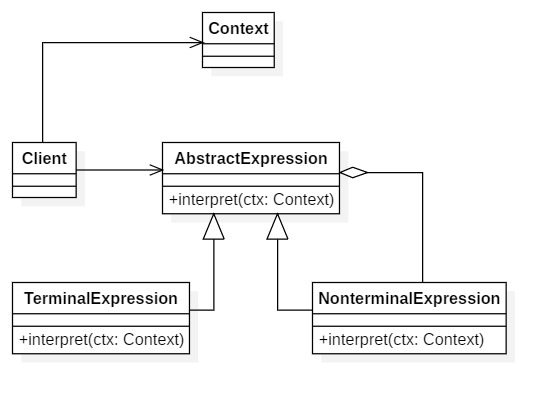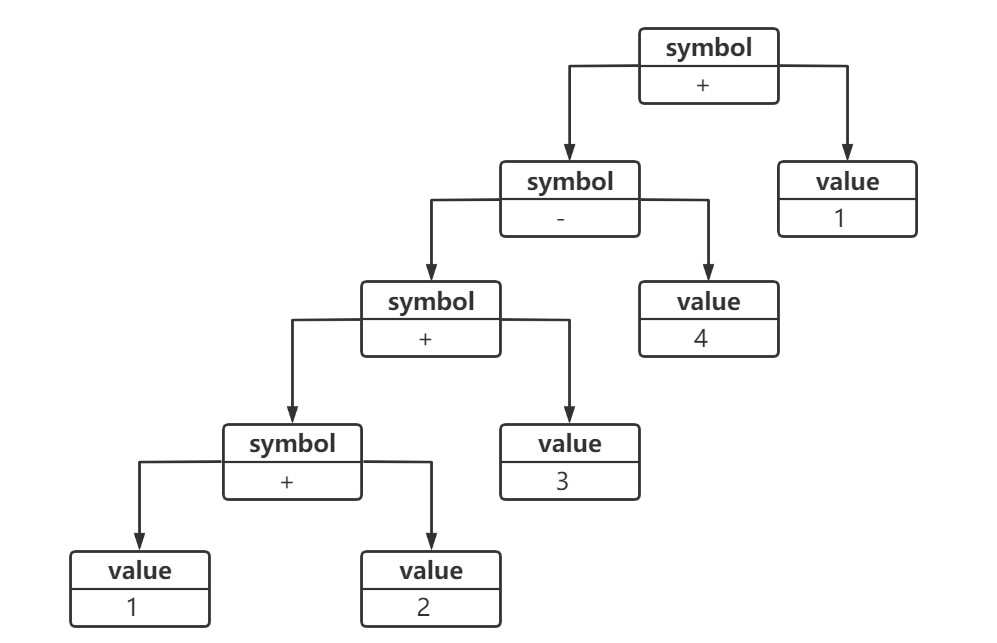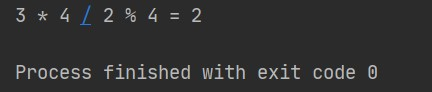# 软件设计模式学习（十九）解释器模式

## 模式结构1. AbstractExpression（抽象表达式）

声明了抽象的解释操作，它是所有终结符表达式和非终结符表达式的公共父类

2. TerminalExpression（终结符表达式）

抽象表达式的子类，实现了文法中的终结符相关联的解释操作，在句子中每一个终结符都是该类的一个实例。

3. NonterminalExpression（非终结符表达式）

也是抽象表达式的子类，实现了文法中的非终结符相关联的解释操作，非终结符表达式中可以包含终结符表达式，也可以继续包含非终结符表达式，因此其解释操作一般通过递归方式来完成。

4. Context（环境类）

环境类又称上下文类，它用于存储解释器之外的一些全局信息，通常它临时存储了需要解释的语句。

5. Client（客户类）

客户类中构造了表示以规定文法定义的一个特定句子的抽象语法树，该抽象语法树由非终结符表达式和终结符表达式实例组合而成。在客户类中还将调用解释操作，实现对句子的解释，有时候为了简化客户类代码，也可以将抽象语法树的构造工作封装到专门的类中完成，客户端只需提供待解释的句子并调用该类的解释操作即可，该类可以称为解释器封装类

## 模式分析

``````expression ::= value | symbol
symbol ::= expression '+' expression | expression '-' expression
value ::= an integer	// 一个整数值
``````

value 是一个终结符表达式，因为它的组成元素就是一个整数值，不能再进行分解。与之对应的 symbol 则是非终结符表达式，它的组成元素仍旧可以是表达式 expression，expression 又可以是 value 或者 symbol，即可以进一步分解。``````public abstract class AbstractExpression {
public abstract void interpret(Context ctx);
}
``````

``````public class TerminalExpression extends AbstractExpression {
public void interpret(Context ctx) {
// 对于终结符表达式的解释操作
}
}

``````

``````public class NonterminalExpression extends AbstractExpression {

private AbstractExpression left;
private AbstractExpression right;

public NonterminalExpression(AbstractExpression left, AbstractExpression right) {
this.left = left;
this.right = right;
}

public void interpret(Context ctx) {
// 递归调用每一个组成部分的 interpret() 方法
// 在递归调用时指定组成部分的连接方式，即非终结符的功能
}
}
``````

``````public class Context {

private HashMap map = new HashMap();

public void assign(String key, String value) {
// 往环境类中设值
}
public void lookup(String key) {
// 获取存储在环境类中的值
}
}
``````

## 模式实例

1. 抽象表达式类 Node（抽象节点）

``````public interface Node {
public int interpret();
}
``````
2. 终结符表达式类 ValueNode（值节点类）

``````public class ValueNode implements Node {

private int value;

public ValueNode(int value) {
this.value = value;
}

@Override
public int interpret() {
return this.value;
}
}
``````
3. 抽象非终结符表达式类 SymbolNode（符号节点类）

``````public abstract class SymbolNode implements Node {

protected Node left;
protected Node right;

public SymbolNode(Node left, Node right) {
this.left = left;
this.right = right;
}
}
``````
4. 非终结符表达式类 MulNode（乘法节点类）

``````public class MulNode extends SymbolNode {

public MulNode(Node left, Node right) {
super(left, right);
}

@Override
public int interpret() {
return super.left.interpret() * super.right.interpret();
}
}
``````
5. 非终结符表达式类 DivNode（除法节点类）

``````public class DivNode extends SymbolNode {

public DivNode(Node left, Node right) {
super(left, right);
}

@Override
public int interpret() {
return super.left.interpret() / super.right.interpret();
}
}
``````
6. 非终结符表达式类 ModNode（求模节点类）

``````public class ModNode extends SymbolNode {

public ModNode(Node left, Node right) {
super(left, right);
}

@Override
public int interpret() {
return super.left.interpret() % super.right.interpret();
}
}
``````
7. 解释器封装类 Calculator（计算器类）

Calculator 类是本实例的核心类之一，Calculator 类中定义了如何构造一棵抽象语法树，在构造过程中使用了栈结构 Stack。通过一连串判断语句判断字符，如果是数字，实例化终结符表达式类 ValueNode 并压栈；如果判断为运算符号，则取出栈顶内容作为其左表达式，而将之后输入的数字封装在 ValueNode 类型的对象作为其右表达式，创建非终结符表达式 MulNode 类型的对象，最后将该表达式压栈。

``````public class Calculator {

private String statement;
private Node node;

public void build(String statement) {

Node left = null, right = null;
Stack<Node> stack = new Stack<Node>();

String[] statementArr = statement.split(" ");

for (int i = 0; i < statementArr.length; i++) {
if (statementArr[i].equalsIgnoreCase(("*"))) {
left = stack.pop();
int val = Integer.parseInt(statementArr[++i]);
right = new ValueNode(val);
stack.push(new MulNode(left, right));
} else if (statementArr[i].equalsIgnoreCase(("/"))) {
left = stack.pop();
int val = Integer.parseInt(statementArr[++i]);
right = new ValueNode(val);
stack.push(new DivNode(left, right));
} else if (statementArr[i].equalsIgnoreCase(("%"))) {
left = stack.pop();
int val = Integer.parseInt(statementArr[++i]);
right = new ValueNode(val);
stack.push(new ModNode(left, right));
} else {
stack.push(new ValueNode(Integer.parseInt(statementArr[i])));
}
}
this.node = stack.pop();
}

public int compute() {
return node.interpret();
}
}
``````
8. 客户端测试类 Client

程序执行时将递归调用每一个表达式类的 interpret() 的解释方法，最终完成对整棵抽象语法树的解释。

``````public class Client {

public static void main(String[] args) {
String statement = "3 * 4 / 2 % 4";
Calculator calculator = new Calculator();
calculator.build(statement);
int result = calculator.compute();
System.out.println(statement + " = " + result);
}
}
``````
9. 运行结果## 模式优缺点

1. 易于改变和扩展文法。由于使用类来表示语言的文法规则，可以通过继承机制来改变或扩展文法。
2. 易于实现文法。抽象语法树中每一个节点类的实现方式都是相似的，编写并不复杂。
3. 增加了新的解释表达式的方式。增加新的表达式时无须对现有表达式类进行修改，符合开闭原则

1. 对于复杂文法难以维护。
2. 执行效率低。解释器模式使用了大量循环和递归调用。
3. 应用场景有限。